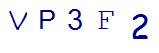# Math Tips, Tricks, and Games

### The 3 Core Principles of Accelerated Learning in K-8 Math

In my quest to create a failure-proof system for learning math, I discovered 3 core principles that shorten...

### Why 7th Grade Algebra Is Challenging For Many Students

Seventh grade is a challenging year for many reasons. Socially, students are in the heart of middle school ...

### 4 Decimal Math Word Problems Your 5th Grader Should Be Able to Solve

Math word problems that include decimals are an important part of 5th-grade math. Students will face more c...

### Why Your Child Gets Stumped by 5th Grade Math Word Problems

Is your child stumped by 5th grade math word problems? Are you dealing with hours of homework and lots of...

### 4th Grade Math Help: 5 Ways to Conquer Factors and Multiples

After kids learn multiplication, a typical 4th grade math curriculum then dives into factors and multiples....

### How to Give Your Child Geometry Help, Teach Proofs like a Math Tutor

Geometry is the branch of mathematics concerned with the properties and relations of points, lines, surface...

### 5 Quick Math Drills to Use with Your 4th Grade Math Student

Fourth grade is a key year for a child’s math education. Granted, every grade in elementary school math is ...

### 8 Math Hacks and Tricks to Turn Your ‘Okay’ Math Student into a Math Champion!

There’s more than one way to solve a math problem.  Even the simple ones!  If you ask your ...

### Tips to Improve Critical Thinking Skills with 1st Grade Math Word Problems

Developing critical thinking skills with math word problems is incredibly important. Students should move b...